×

Search anything:

# Queue in C++ STL

#### C++ Software Engineering standard template library queueThe queue in the STL of C++ is a dynamically resizing container to implement the queue data structure. It is a sequential linear container which follows the First In First Out(FIFO) arrangement.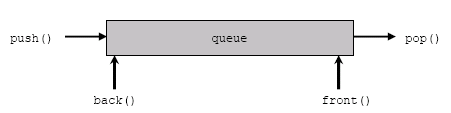### Definition in `<queue>`

std::queue is defined as follows under `<queue>` header file:

``````template <class T, class Container = deque<T> > class queue;
``````

### Declaration

A queue with name a storing integers is declared as follows:

``````queue<int> a;
``````

### Functions

Assume the queue a to be: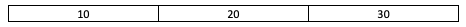and the queue b to be: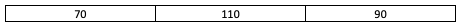The following functions are defined under the `<queue>` header file:

### Illustration of Queue

``````// C++ code to illustrate queue in Standard Template Library (STL)
#include <iostream>
#include <queue>

using namespace std;

void display(queue <int> q)
{
queue <int> c = q;
while (!c.empty())
{
cout << " " << c.front();
c.pop();
}
cout << "\n";
}

int main()
{
queue <int> a;
a.push(10);
a.push(20);
a.push(30);

cout << "The queue a is :";
display(a);

cout << "a.empty() :" << a.empty() << "\n";
cout << "a.size() : " << a.size() << "\n";
cout << "a.front() : " << a.front() << "\n";
cout << "a.back() : " << a.back() << "\n";

cout << "a.pop() : ";
a.pop();
display(a);

a.push(40);
cout << "The queue a is :";
display(a);

return 0;
}
``````
``````\\Console output
The queue a is : 10 20 30
a.empty() :0
a.size() : 3
a.front() : 10
a.back() : 30
a.pop() :  20 30
The queue a is : 20 30 40
``````
###### State of Queue a after each iteration: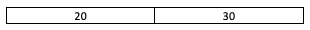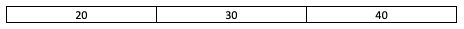### Question

#### Choose the correct option

queue has fixed size and it overflows upon exceeding maximum size
queue is dynamically resized based on its contents
Queue is a container which is dynamically resized whenever a push/pop operation is performed.